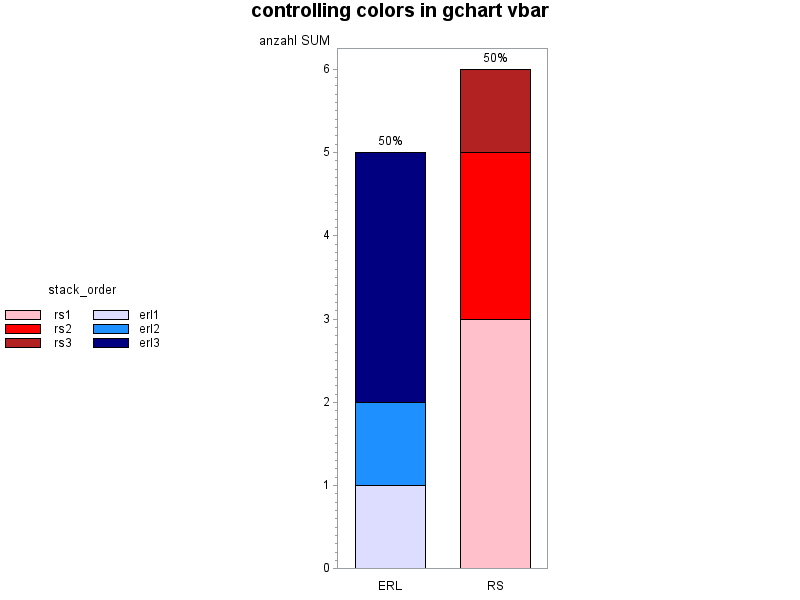## Question coloring VBars of Midpoints

Dear all,

I'm a very new graphics-user and I hope my question is not too boring for you:

I have a proc gchart

legend1 frame
label=("Prio:" position=top justify=center)
position=(middle right outside)
mode=protect
across=1;

proc gchart data= tmp_Grafikdaten_X (where=(RS_ERL in( "RS", "ERL") )) ;
format anzahl 8. ;
format Datum date9.;
VBAR RS_ERL /
sumvar = Anzahl
TYPE=SUM
midpoints="RS" "ERL"
group=datum
subgroup=kattxt
legend=legend1
RAXIS=AXIS1
MAXIS=AXIS2
GAxis=axis3

;

and I would like to color the bars of the 2 midpoints in different color schemes. For example the subgroups of midpoint RS should be shown in blue tones and midpoint ERL should be shown in red tones.

Is this possible?

And if it is possible, how  can I output the legend accordingly?

Many thanks for all hints!

1 ACCEPTED SOLUTION

Accepted Solutions

## Re: Question coloring VBars of Midpoints

It's a bit 'cumbersome' matching specific colors to specific bar segments in gchart, but here's one way to do it...

(assuming you have the exact same number of bar segments each time you run the graph)

data tmp_grafikdaten_x;
length rs_erl \$5;
length kattxt \$10;
input rs_erl stack_order kattxt anzahl;
datalines;
RS 1 rs1 3
RS 2 rs2 2
RS 3 rs3 1
ERL 4 erl1 1
ERL 5 erl2 1
ERL 6 erl3 3
;
run;

proc sql noprint;
create table control as
select unique stack_order as start, kattxt as label
from tmp_grafikdaten_x;
quit; run;
data control; set control;
fmtname='stkfmt';
type='N';
run;proc format lib=work cntlin=control;
run;

legend1 position=(middle left) across=2 mode=share
label=(position=top justify=center) colmajor;

pattern1 v=s c=pink;
pattern2 v=s c=red;
pattern3 v=s c=firebrick;
pattern4 v=s c=cxddddff;
pattern5 v=s c=dodgerblue;
pattern6 v=s c=navy;

axis1 label=none;

title "controlling colors in gchart vbar";
proc gchart data= tmp_Grafikdaten_X (where=(RS_ERL in( "RS", "ERL") )) ;
format stack_order stkfmt.;
vbar rs_erl / type=sum sumvar=anzahl legend=legend1
subgroup=stack_order width=10pct space=5pct maxis=axis1;
run;4 REPLIES 4

## Re: Question coloring VBars of Midpoints

Without some actual data I wouldn't promise it is possible with GCHART.
As a minimum that can involve a lot of Symbol statements that are dependent on data value order.

Proc SGPLOT has an option DATTRMAP that lets you set characteristics per formatted value of a Group variable and would be where I was going.

## Re: Question coloring VBars of Midpoints

Thank you for the hint. But there is no subgroup-option, isn't it?

## Re: Question coloring VBars of Midpoints

It's a bit 'cumbersome' matching specific colors to specific bar segments in gchart, but here's one way to do it...

(assuming you have the exact same number of bar segments each time you run the graph)

data tmp_grafikdaten_x;
length rs_erl \$5;
length kattxt \$10;
input rs_erl stack_order kattxt anzahl;
datalines;
RS 1 rs1 3
RS 2 rs2 2
RS 3 rs3 1
ERL 4 erl1 1
ERL 5 erl2 1
ERL 6 erl3 3
;
run;

proc sql noprint;
create table control as
select unique stack_order as start, kattxt as label
from tmp_grafikdaten_x;
quit; run;
data control; set control;
fmtname='stkfmt';
type='N';
run;proc format lib=work cntlin=control;
run;

legend1 position=(middle left) across=2 mode=share
label=(position=top justify=center) colmajor;

pattern1 v=s c=pink;
pattern2 v=s c=red;
pattern3 v=s c=firebrick;
pattern4 v=s c=cxddddff;
pattern5 v=s c=dodgerblue;
pattern6 v=s c=navy;

axis1 label=none;

title "controlling colors in gchart vbar";
proc gchart data= tmp_Grafikdaten_X (where=(RS_ERL in( "RS", "ERL") )) ;
format stack_order stkfmt.;
vbar rs_erl / type=sum sumvar=anzahl legend=legend1
subgroup=stack_order width=10pct space=5pct maxis=axis1;
run;## Re: Question coloring VBars of Midpoints

Thanks a lot, this is exactly what I wanted!

Discussion stats
• 4 replies
• 324 views
• 1 like
• 3 in conversation